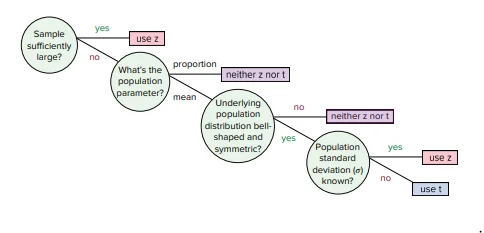27 Mar 2023

Posted on:

31 Jan 2023

0

# Resolved:Z-statistic and t-statistic

What if the population variance is unknown and the sample is big. What statistic would we be using? Because from the exmaples illustrated - we use t-test for unknown and small and z-test for known and big

Posted on:

01 Feb 2023

0

If the sample is bigger than 30 (n>30 for mean and n>15 for proportions) the CLT (central limit theorem) comes into play and you can use z-statistic. If you do not know the population variance, you use the sample variance.

Posted on:

01 Feb 2023

0

Thank you for addressing my question. What do you mean by proportions in this context?

Posted on:

02 Feb 2023

0
Posted on:

07 Mar 2023

0

i dont agree with his answer because we only use CLT when we have many samples with different sizes so then we take mean for all of them and create a distribution of those means called sampling distribution and to answer your question we would use the z statistic.

So, to summarize:

If the population variance is known and the sample size is large or small, we can use a z-test.
If the population variance is unknown and the sample size is small (less than 30), we should use a t-test.
If the population variance is unknown and the sample size is large (greater than 30), we can use a z-test.

Posted on:

27 Mar 2023

0Posted on:

27 Mar 2023

0

for small samples, you can follow this decision tree above, For larger samples CLT will apply so you can use z-statistic